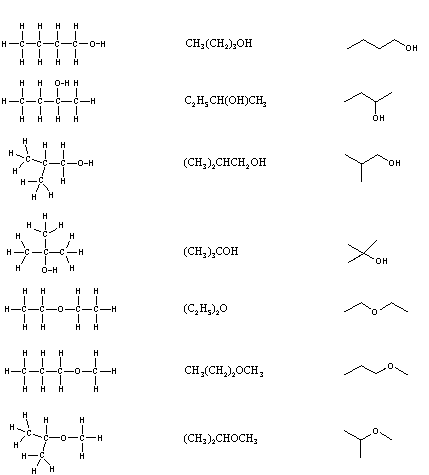# 1.7: Drawing Organic Structures

$$\newcommand{\vecs}{\overset { \rightharpoonup} {\mathbf{#1}} }$$ $$\newcommand{\vecd}{\overset{-\!-\!\rightharpoonup}{\vphantom{a}\smash {#1}}}$$$$\newcommand{\id}{\mathrm{id}}$$ $$\newcommand{\Span}{\mathrm{span}}$$ $$\newcommand{\kernel}{\mathrm{null}\,}$$ $$\newcommand{\range}{\mathrm{range}\,}$$ $$\newcommand{\RealPart}{\mathrm{Re}}$$ $$\newcommand{\ImaginaryPart}{\mathrm{Im}}$$ $$\newcommand{\Argument}{\mathrm{Arg}}$$ $$\newcommand{\norm}{\| #1 \|}$$ $$\newcommand{\inner}{\langle #1, #2 \rangle}$$ $$\newcommand{\Span}{\mathrm{span}}$$ $$\newcommand{\id}{\mathrm{id}}$$ $$\newcommand{\Span}{\mathrm{span}}$$ $$\newcommand{\kernel}{\mathrm{null}\,}$$ $$\newcommand{\range}{\mathrm{range}\,}$$ $$\newcommand{\RealPart}{\mathrm{Re}}$$ $$\newcommand{\ImaginaryPart}{\mathrm{Im}}$$ $$\newcommand{\Argument}{\mathrm{Arg}}$$ $$\newcommand{\norm}{\| #1 \|}$$ $$\newcommand{\inner}{\langle #1, #2 \rangle}$$ $$\newcommand{\Span}{\mathrm{span}}$$$$\newcommand{\AA}{\unicode[.8,0]{x212B}}$$

It is necessary to draw structural formulas for organic compounds because in most cases a molecular formula does not uniquely represent a single compound. Different compounds having the same molecular formula are called isomers, and the prevalence of organic isomers reflects the extraordinary versatility of carbon in forming strong bonds to itself and to other elements. When the group of atoms that make up the molecules of different isomers are bonded together in fundamentally different ways, we refer to such compounds as constitutional isomers. There are seven constitutional isomers of C4H10O, and structural formulas for these are drawn in the following table. These formulas represent all known and possible C4H10O compounds, and display a common structural feature. There are no double or triple bonds and no rings in any of these structures.

Structural Formulas for C4H10O isomers

 Kekulé Formula Condensed Formula Line-bond (or skeletal) FormulaSimplification of structural formulas may be achieved without any loss of the information they convey. In condensed structural formulae the bonds to each carbon are omitted, but each distinct structural unit (group) is written with subscript numbers designating multiple substituents, including the hydrogens. Line-bond (skeletal) formulae omit the symbols for carbon and hydrogen entirely. Each straight line segment represents a bond, the ends and intersections of the lines are carbon atoms, and the correct number of hydrogens is calculated from the tetravalency of carbon. Non-bonding valence shell electrons are omitted in these formulas.

Developing the ability to visualize a three-dimensional structure from two-dimensional formulas requires practice, and in most cases the aid of molecular models. As noted earlier, many kinds of model kits are available to students and professional chemists, and the beginning student is encouraged to obtain one.

### Kekulé Formulae

A structural formula displays the atoms of the molecule in the order they are bonded. It also depicts how the atoms are bonded to one another, for example single, double, and triple covalent bond. Covalent bonds are shown using lines. The number of dashes indicate whether the bond is a single, double, or triple covalent bond. Structural formulas are helpful because they explain the properties and structure of the compound which empirical and molecular formulas cannot always represent.

Ex. Kekulé Formula for Ethanol:### Condensed Formulae

Condensed structural formulas show the order of atoms like a structural formula but are written in a single line to save space and make it more convenient and faster to write out. Condensed structural formulas are also helpful when showing that a group of atoms is connected to a single atom in a compound. When this happens, parenthesis are used around the group of atoms to show they are together.

Ex. Condensed Structural Formula for Ethanol: CH3CH2OH (Molecular Formula for Ethanol C2H6O).

### Line-bond (Skeletal) Formulae

Because organic compounds can be complex at times, line-angle formulas are used to write carbon and hydrogen atoms more efficiently by replacing the letters with lines. A carbon atom is present wherever a line intersects another line. Hydrogen atoms are then assumed to complete each of carbon's four bonds. All other atoms that are connected to carbon atoms are written out. Line angle formulas help show structure and order of the atoms in a compound making the advantages and disadvantages similar to structural formulas.

Ex.Skeletal Formula for Ethanol:## Contributors

• Jean Kim (UCD), Kristina Bonnett (UCD)
• Layne Morsch (University of Illinois Springfield)

This page titled 1.7: Drawing Organic Structures is shared under a CC BY-NC-SA 4.0 license and was authored, remixed, and/or curated by Layne Morsch.